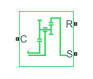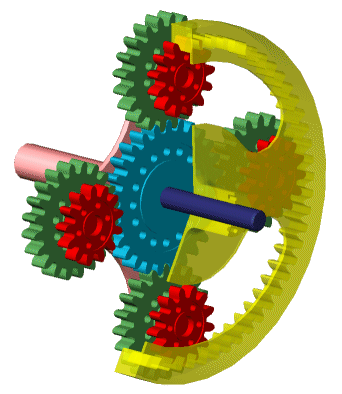# Compound Planetary Gear

Planetary gear train with stepped planet gear set

• Library:
• Simscape / Driveline / Gears

•## Description

The Compound Planetary Gear block represents a planetary gear train with composite planet gears. Each composite planet gear is a pair of rigidly connected and longitudinally arranged gears of different radii. One of the two gears engages the centrally located sun gear while the other engages the outer ring gear.

Compound Planetary GearThe block models the compound planetary gear as a structural component based on the Simscape™ Driveline™ Sun-Planet and Ring-Planet blocks. The figure demonstrates the equivalent block diagram for the Compound planetary gear block.To increase the fidelity of the gear model, specify properties such as gear inertia, meshing losses, and viscous losses. By default, gear inertia and viscous losses are assumed to be negligible. The block enables you to specify the inertias of the internal planet gears. To model the inertias of the carrier, sun, and ring gears, connect Simscape Inertia blocks to ports C, S, and R.

### Thermal Model

You can model the effects of heat flow and temperature change by enabling the optional thermal port. To enable the port, set Friction model to ```Temperature-dependent efficiency```.

### Equations

Ideal Gear Constraints and Gear Ratios

The Compound Planetary Gear block imposes two kinematic and two geometric constraints.

`${r}_{C}{\omega }_{C}={r}_{S}{\omega }_{S}+{r}_{P1}{\omega }_{P},$`

`${r}_{R}{\omega }_{R}={r}_{C}{\omega }_{C}+{r}_{P2}{\omega }_{P},$`

`${r}_{C}={r}_{S}+{r}_{P1},$`

`${r}_{R}={r}_{C}+{r}_{P2},$`

where:

• rC is the radius of the carrier gear.

• ωC is the angular velocity of the carrier gear.

• rS is the radius of the sun gear.

• ωS is the angular velocity of the sun gear.

• rP1 is the radius of planet gear 1.

• ωP is the angular velocity of the planet gears.

• rP2 is the radius of planet gear 2.

• rR is the radius of the ring gear.

The ring-planet and planet-sun gear ratios are:

`${g}_{RP}={r}_{R}/{r}_{P2}={N}_{R}/{N}_{P2}$`

and

`${g}_{PS}={r}_{P1}/{r}_{S}={N}_{P1}/{N}_{S},$`

where:

• gRP is the ring-planet gear ratio.

• NR is the number of teeth on the ring gear.

• NP2 is the number of teeth on planet gear 2.

• gPS is the planet-sun gear ratio.

• NP1 is the number of teeth on planet gear 1.

• NS is the number of teeth on the sun gear.

In terms of the gear ratios, the key kinematic constraint is:

The four degrees of freedom reduce to two independent degrees of freedom. The gear pairs are (1, 2) = (P2, R) and (S, P1).

Warning

The gear ratio gRP must be strictly greater than one.

The torque transfers are:

and

where:

• τP2 is torque transfer for planet gear 2.

• τR is torque transfer for the ring gear.

• τloss is torque transfer loss.

• τS is torque transfer for the sun gear.

• τP1 is torque transfer for planet gear 1.

In the ideal case where there is no torque loss, τloss = 0.

Nonideal Gear Constraints and Losses

In the nonideal case, τloss ≠ 0. For more information, see Model Gears with Losses.

### Assumptions and Limitations

• Gear inertia is assumed to be negligible.

• Gears are treated as rigid components.

## Ports

### Conserving

expand all

Rotational mechanical conserving port associated with the planet gear carrier.

Rotational mechanical conserving port associated with the ring gear.

Rotational mechanical conserving port associated with the sun gear.

Thermal conserving port associated with heat flow. Heat flow affects the power transmission efficiency by altering the gear temperatures.

#### Dependencies

To enable this port, set Friction model to `Temperature-dependent efficiency`.

## Parameters

expand all

### Main

Fixed ratio, gRP, of the ring gear to the planet gear rotations as defined by the number of ring gear teeth divided by the number of planet gear teeth. This gear ratio must be strictly greater than `1`.

Fixed ratio, gPS, of the planet gear to the sun gear rotations as defined by the number of planet gear teeth divided by the number of sun gear teeth. This gear ratio must be strictly greater than `0`.

### Meshing Losses

Friction model for the block:

• `No meshing losses - Suitable for HIL simulation` — Gear meshing is ideal.

• `Constant efficiency` — Transfer of torque between the gear wheel pairs is reduced by a constant efficiency, η, such that 0 < η ≤ 1.

• `Temperature-dependent efficiency` — Transfer of torque between the gear wheel pairs is defined by the table lookup based on the temperature.

Vector of torque transfer efficiencies, [ηSP ηRP], for sun-planet and ring-carrier gear wheel pair meshings, respectively. The vector element must be in the interval (0,1].

#### Dependencies

To enable this parameter, set Friction model to ```Constant efficiency```.

Vector of temperatures used to construct a 1-D temperature-efficiency lookup table. The vector elements must increase from left to right.

#### Dependencies

To enable this parameter, set Friction model to `Temperature-dependent efficiency`.

Vector of output-to-input power ratios that describe the power flow from the sun gear to the planet gear, ηSP. The block uses the values to construct a 1-D temperature-efficiency lookup table.

Each element is an efficiency that relates to a temperature in the Temperature vector. The length of the vector must be equal to the length of the Temperature vector. Each element in the vector must be in the range (0,1].

#### Dependencies

To enable this parameter, set Friction model to ```Temperature-dependent efficiency```.

Vector of component efficiencies, ηRP—the ratio of output power to input power, that the block uses to construct a 1-D temperature-efficiency lookup table.

Each element is an efficiency that relates to a temperature in the Temperature vector. The length of the vector must be equal to the length of the Temperature vector. Each element in the vector must be in the range (0,1].

#### Dependencies

To enable this parameter, set Friction model to ```Temperature-dependent efficiency```.

Vector of power thresholds above which the full efficiency factors apply. Enter the thresholds in the order sun-carrier, planet-carrier. Below these values, a hyperbolic tangent function smooths the efficiency factor.

When you set Friction model to `Constant efficiency`, the block lowers the efficiency losses to zero when no power is transmitted. When you set Friction model to `Temperature-dependent efficiency`, the block smooths the efficiency factors between zero when at rest and the values provided by the temperature-efficiency lookup tables at the power thresholds.

#### Dependencies

To enable this parameter, set Friction model to `Constant efficiency` or ```Temperature-dependent efficiency```.

### Viscous Losses

Vector of viscous friction coefficients, [μS μP], for the sun-carrier and planet-carrier gear motions, respectively.

### Inertia

Inertia model for the block:

• `Off` — Model gear inertia.

• `On` — Neglect gear inertia.

Moment of inertia of the combined planet gears. This value must be positive.

#### Dependencies

To enable this parameter, set Inertia to `On`.

### Thermal Port

To enable this parameter, set Friction model to `Temperature-dependent efficiency`.

Thermal energy required to change the component temperature by a single temperature unit. The greater the thermal mass, the more resistant the component is to temperature change.

#### Dependencies

To enable this parameter, set Friction model to ```Temperature-dependent efficiency```.

Block temperature at the start of simulation. The initial temperature sets the initial component efficiencies according to their respective efficiency vectors.

#### Dependencies

To enable this parameter, set Friction model to `Temperature-dependent efficiency`.

expand all

## Version History

Introduced in R2011a